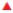# The energies of the orbitals in many elements have been determined. For the first two periods they have the following values: (a) Why do the orbital energies generally become more negative on proceeding across the second period? (b) How are these values related to the ionization energy and electron attachment enthalpy of the elements? (c) Use these energy values to explain the observa­tion that the ionization energies of the first four second-period elements are in the order Li &lt; Be &gt; B &lt; C. (Data from J. B. Mann, T. L Meek, and L C. Allen: Journal of the American Chemical Society, Vol. 122, p. 2780. 2000.)### Chemistry & Chemical Reactivity

9th Edition
John C. Kotz + 3 others
Publisher: Cengage Learning
ISBN: 9781133949640

#### Solutions

Chapter
Section### Chemistry & Chemical Reactivity

9th Edition
John C. Kotz + 3 others
Publisher: Cengage Learning
ISBN: 9781133949640
Chapter 7, Problem 75SCQ
Textbook Problem
42 views

## The energies of the orbitals in many elements have been determined. For the first two periods they have the following values:(a) Why do the orbital energies generally become more negative on proceeding across the second period? (b) How are these values related to the ionization energy and electron attachment enthalpy of the elements? (c) Use these energy values to explain the observa­tion that the ionization energies of the first four second-period elements are in the order Li < Be > B < C. (Data from J. B. Mann, T. L Meek, and L C. Allen: Journal of the American Chemical Society, Vol. 122, p. 2780. 2000.)

a)

Interpretation Introduction

Interpretation:

The orbital energies become more negative on proceeding across the second period, the reason has to be discussed.

Concept Introduction:

Ionization energy (IE): The ionization energy is the required to remove an electron from an atom in the gas phase.

General formula of ionization energy= Atom in the ground state(g)Atom+(g)+eΔU=Ionizationenergy(IE)

As predicted by coulomb’s law, energy must be supplied to overcome the attraction between an electron and the nucleus and to separate the electron from the atom. Thus ionization energies always have positive values. An electron father from the nucleus generally has smaller ionization energy and electron closer to the nucleus has larger ionization energy.

Increase and decrease electro negativity: The less vacancy electrons an atoms has the least it will gain of electrons. Moreover the electron affinity decrease down the groups and from right to left across the periods on the periodic table, the reason is electrons are placed in a higher energy level far from the nucleus thus a decrease from its pull.

### Explanation of Solution

Along the period the effective nuclear charge increases, that pulls the valence electrons internally and ...

b)

Interpretation Introduction

Interpretation:

The given value has to be related with ionization energy and electron attachment enthalpy of the elements.

Concept Introduction:

Ionization energy (IE): The ionization energy is the required to remove an electron from an atom in the gas phase.

General formula of ionization energy= Atom in the ground state(g)Atom+(g)+eΔU=Ionizationenergy(IE)

As predicted by coulomb’s law, energy must be supplied to overcome the attraction between an electron and the nucleus and to separate the electron from the atom. Thus ionization energies always have positive values. An electron father from the nucleus generally has smaller ionization energy and electron closer to the nucleus has larger ionization energy.

Increase and decrease electro negativity: The less vacancy electrons an atoms has the least it will gain of electrons. Moreover the electron affinity decrease down the groups and from right to left across the periods on the periodic table, the reason is electrons are placed in a higher energy level far from the nucleus thus a decrease from its pull.

c)

Interpretation Introduction

Interpretation:

The observation that the ionization energies of elements in the order Li < Be > B < C has to be explained.

Concept Introduction:

Ionization energy (IE): The ionization energy is the required to remove an electron from an atom in the gas phase.

General formula of ionization energy = Atom in the ground state(g)Atom+(g)+eΔU=Ionizationenergy(IE)

As predicted by coulomb’s law, energy must be supplied to overcome the attraction between an electron and the nucleus and to separate the electron from the atom. Thus ionization energies always have positive values. An electron father from the nucleus generally has smaller ionization energy and electron closer to the nucleus has larger ionization energy.

Increase and decrease electro negativity: The less vacancy electrons an atoms has the least it will gain of electrons. Moreover the electron affinity decrease down the groups and from right to left across the periods on the periodic table, the reason is electrons are placed in a higher energy level far from the nucleus thus a decrease from its pull.

### Still sussing out bartleby?

Check out a sample textbook solution.

See a sample solution

#### The Solution to Your Study Problems

Bartleby provides explanations to thousands of textbook problems written by our experts, many with advanced degrees!

Get Started

Find more solutions based on key concepts
What are the moral implications of using ergogenic aids?

Understanding Nutrition (MindTap Course List)

In high doses, tryptophan can improve nausea and skin disorders. T F

Nutrition: Concepts and Controversies - Standalone book (MindTap Course List)

How does an ionic bond form?

Biology: The Dynamic Science (MindTap Course List)

Where is oceanic primary productivity highest?

Oceanography: An Invitation To Marine Science, Loose-leaf Versin

A right circular cone can theoretically be balanced on a horizontal surface in three different ways. Sketch the...

Physics for Scientists and Engineers, Technology Update (No access codes included)